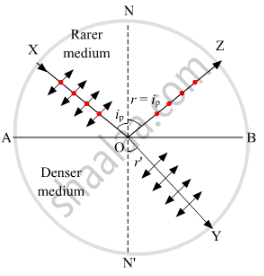# Derive the Expression of Brewster'S Law When Unpolarised Light Passing from a Rarer to a Denser Medium Gets Polarised on Reflection at the Inteface. - Physics

Derive the expression of Brewster's law when unpolarised light passing from a rarer to a denser medium gets polarised on reflection at the inteface.

#### SolutionLet us consider that an unpolarised light is incident along XO at an angle ip(angle of polarisation) on the interface AB, separating air, a rarer medium, from a denser medium of refractive index μ. It has been experimentally observed that when unpolarised light is incident at polarising angle, the reflected components along OZ and OY are mutually perpendicular to each other. So, from the above figure, we have

ZOB+BOY=90°

(90°ip)+(90°r')=90°

where r' is the angle of refraction

90°ip=r'

According to Snell's law,

μ=sini/sinr

Here,

i=ip

r=r'=(90°ip)

∴μ="sinip"/(sin(90°−ip))

⇒μ="sinip"/"cosip"=tanip

This gives us the required expression for Brewster's law.

Concept: Brewster's Law
Is there an error in this question or solution?